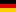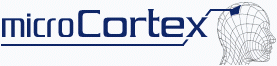Click here for linear version of these pages.New Account
News
Why ANN?
To Get Started
WalkThrough Guide
Guide: Credit Risk Assessment
Guide: How to Use and Features
 Guide: How to Analyse the ANN
Data Types
Very Quick Guide to Neural Networks
ANN: More DetailsPrivacy Statement
Find at our Site
Impressum

## 1. Introduction

 You will understand how to: read the regression graph (predicted vs. observed values) read the sensitivity graph (sensitivity of the ouput on the input)
This is demonstrated on the diagnosis of resistant bacteria in human.

## 2. Generation of the Data-Set Top

The data is totally artificial. However, we tried to base it on a model that should reflect somewhat the reality. Since one important feature discussed here is about the underlying modell to the data, we don't uncover too much here. The input data (the independent variables) categories are:
• Treatment previous 3 months 0/1
• Last treatment ended [month]
• Last treatment duration [days]
• Otitis-prone condition 0/1
• Time since last treated ear infection [months]
• Daycare attendance 0/1
• Age [years]
• Time since last hospitalization [month]
• randomA
• randomB
• randomC
The output data (the dependent variables) categories are:
(scaled between 0 - 1000)
• Specific resistance [au]
• Unspecific resistance [au]
Things we have to reveal:
• "Treatment previous 3 months" is calculated based on "Last treatment ended".
• The values of the remaining columns are generated based on random number, scaled to the desired range.
• "Last treatment duration" is tuned to be best at 5 days.
• "randomA", "randomB" and "randomC" are random numbers between 0 and 1 (you have to you know this in order to provide the right values for predictions, however, it is irrelevant for the ANN, so it could bee scaled from 2.5 to 7.8) which the output does not depend on.
• The output is calculated with a different formula for "Specific resistance" and "Unspecific resistance", those three "randomA", "randomB" and "randomC" columns are never taken into account.
The data-set consists of 873 cases.

Yes, there is more too say, No, we don't reveal it now, please read on...

## 3. Analysis of the ANN TopOn the left upper field of the "ANN Analysis/Trained ANN Statistics" page you find statistical key values for the ANN presented as text, as shown in the figure on the left. A more complete report is available by clicking on the links "text format report".
Now, if you have more than one output, like in this example, all the information shown referes to the selected output (the first one is selected by default). The "Predicted vs. Observed Regression Graph" has the name of the selected output in the title.
If you request the text report, you have to force a reload in your browser to see the actual report and not the previous one from the previously selected output.

So here you got:

• r2=0.99834, which means that the ANN predicts quite well.
• 11 inputs with 10 optimal hidden nodes, which means that either the problem is complex or the ANN has only learned by heart. The latter one would mean that predictions might not be very good on cases that weren't seen before.
In order to get a new analysis, select the output for which you want the analysis for and then press "New Output Analysis".

## 4. Regression Analysis Top

Elements of the "Predicted vs. Observed Regression Graph".
• open circles: values used for training the selected ANN, the one that you are going to use. It is the ANN which is the least biased, as determined by the cross validation procedure. In other words, the ANN which is best suited.
• crosses: values used for testing the selected ANN (these values were not used in the training of the elected/best ANN!)
• dashed line: represents the optimal case, where the predicted values are equal the observed values
• solid line: linear regression of the testing values (the closer this line is to the dashed (ideal) line, the better)
Let's look at three different "pictures":
• Poor distribution of data
• Poor regression
• Good regression
We start with the "poor distribution of data":The gathering around 0 and around 1 is not necessarelly bad, if you wanted a "yes/no" answer. However, you should submit a data-set which has an almost equal number of values at 0 and at 1. Since this is not the case, the quality of the ANN is probably not OK. Although the regression is not far from the ideal line (dashed), you should consider making a new selection of your data and resubmit it.This kind of graph is not suitable for frequency analysis. So, you should use this graph to decide that you have to make a frequency analysis. You might have enough values around zero, but you can't tell it by this graph.
The point is, there are too many values too far away from from the regression line.

Again, don't trust this ANN.This both graphs point to a good quality ANN.

1. No visible difference between ideal and observed regression line.
2. Aparently good distribution of the vaules over the range of interest.
3. Small number of values far away from the ideal regression.

## 5. Sensitivity Analysis TopWhat strikes immediatly is that input number 3 (Last treatment duration) is THE factor which influences the output (Specific Resistance). The other inputs have little influence.
In the real world, you would stop here. But since we know how the input data was generated, we can tell more about the ANN. And since this is for educational purpose, this is the right thing to do.

The last three columns are random number, hence they had no influence on the output. So inputs that show a similar level of influence on the output, should be considered irrelevant.
So, either the formula use to generate the data is bad or the ANN or both. Well, taking the regression analysis into account, at least the ANN has to be considered bad. But, looking at a frequency analysis of the output data, there is also a problem here: not enought values around "1000".For the unspecific resistance, the big picture is the same. Inputs 7 (Age) and 8 (Time since last hospitalization) are a little bit more above the noise. But not convincing.

In the real world, you would have selected the data to give a balanced distribution of the output values, with respect to the type of prediction you want.
In this case, this would be a distribution balanced between 0 and 1000, and not something like, 10% of the values between 0 and 100, 80% between 900 and 1000, or vice versa.

Now to ANNs with more power:Please disregard the blue bars for now, we will explain them later on.
So inputs 1 - 4 and 6 are relevant for the output (now: specific resistance), the others are irrelevant.
Input 3 is dominant.Now we look at the unspecific resistance.
There are differences in the pattern. However, the dominance of input 3 is here too. But let's look at the differences.

The relevant inputs are now 2, 3, 6 to 8. 1 and 4 lost relevance, 7 and 8 gained relevance.
Looking at the noise, we can suspect that there is significant difference in the way specific and unspecific resistance is acquired.

Now to the different two bars:

• the red one shows the sensitivity of the output you selected to the respective input
• the blue one shows the sensitivity of all outputs to the respective inputThe figure on the left tells us that input 1 has influence on the selected output (Specific Resistance) above average. The red bar is considerable higher than the blue one.Compared to the "Specific Resistance", input 1 has lost its influence and has to be considered irrelevant for the "unspecific resistance". So we are able to point out different key factors for the two types of resistances and this is helpful in creating a modell on what is important to avoid antibiotic resistances.